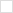This wiki is locked. Future workgroup activity and specification development must take place at our new wiki. For more information, see this blog post about the new governance model and this post about changes to the website.
TWiki>Main Web>PmHome>MetricsHome>MetricsWorkingDocuments>MetricsEMS10RestApi>MetricsEMS10RestApiDataModel>MetricsEmsQuantileFunction (24 Sep 2010, ArthurRyman)

# ems:QuantileFunction

Probability distributions: ems:PointEstimate, ems:UniformDistribution, ems:TriangularDistribution, ems:NormalDistribution, ems:PoissonDistribution, ems:QuantileFunction, ems:CumulativeDistributionFunction

## Description

Although some important probability distributions can be represented by simple analytic formulas (e.g. ems:UniformDistribution, ems:TriangularDistribution, ems:NormalDistribution, and ems:PoissonDistribution), in general the result of an estimation run will be an arbitrary function which can only be approximated numerically. A quantile function is a very useful way of approximately representing any probability distribution to any desired degree of accuracy. A quantile function is given by dividing the range of probabilities into some given number of equal intervals, and specifying the values for which the cumulative probability reaches the probability for each interval.

Each probability range is called a quantile. Some quantiles are given special names for commonly used numbers of intervals. For example, quantiles are called quartiles when the number of equal intervals is 4, deciles when 10, and percentiles when 100. For example, a project duration estimate may be expressed as a quartile function by specifying that there is a 0.25 probability that the project will take no longer than 6 months, a 0.50 probability for 8 months and a 0.75 probability for 9 months.

The number of quantiles must be greater than 1 since if there is only 1 interval we have a ems:UniformDistribution. The number of quantile values given is 1 less than the number of quantiles, i.e. we do not assume the probability distribution is bounded. If the probability distribution has low or high bounds, we describe them as follows.

In general, a probability distribution may have non-zero values for arbitrarily small or large values, i.e. the cumulative probability may only reach 0 at negative infinity and 1 at positive infinity. However, for software estimates, the range of possible values is finite in practice and it is useful to include these low and high values in the representation of the quantile function. For example, in a percentile function, the 0th percentile value is the low value, i.e. no value falls below it, and the 100th percentile is the high value, i.e. no value falls above it. In the preceding example, it may be impossible that the project will take less than 5 months, and certain that the project will complete within 12 months.

An ems:QuantileFunction resource represents a quantile function.

## Properties

Property Range Type Occurrence Edits Description
ems:numberOfQuantiles datatype xsd:int exactly-one read-write The number of quantiles, e.g. 4 for quartiles.
ems:low datatype xsd:double exactly-one read-write The low value.
ems:quantile local inline resource ems:Quantile one-or-more read-write A quantile value.
ems:high datatype xsd:double exactly-one read-write The high value.

### ems:numberOfQuantiles

This required property is the number of quantiles, e.g. 4 for quartiles, 100 for percentiles. It MUST be greater than 1.

### ems:low

This required property is the low value of the distribution. The probability is 0 below this value and goes positive above it.

### ems:quantile

This required, multi-valued property gives inline ems:Quantile resources which specify the cumulative probability of each quantile and its value. The number of these properties MUST be one less than the number of quantiles and they MUST define all the quantile probabilities between 0 (low) and 1 (high). Each quantile value MUST be greater than the low value and less than the high value, and they MUST define a non-descending sequence when ordered by cumulative probability.

### ems:high

This required property is the high value of the distribution. The probability is 1 above this value and goes less than 1 below it.

## ems:Quantile

This inline resource represents the value of the distribution for a given quantile probability.

It has the following properties:

Property Range Type Occurrence Edits Description
ems:probability datatype xsd:double exactly-one read-write The cumulative probability of this quantile.
ems:numericValue datatype xsd:double exactly-one read-write The value of the distribution for this quantile.

### ems:probability

This required property is the cumulative probability of this quantile. For example, in a quartile function the cumulative probabilities are .25, .50, and .75.

### ems:numericValue

This required property is the value for which the cumulative probability of this quantile is attained, i.e. the probability that a measurement gives a result less than or equal to this value is equal to the cumulative probability of this quantile.

## Examples

### Quantile Function 1

Consider the following table of quartile cumulative probabilities:

probability value
0.00 18
0.25 20
0.50 25
0.75 35
1.00 50

This quartile function has the following representation as an ems:QuantileFunction resource:

###### Listing of Quantile Function 1
```<?xml version="1.0"?>
<ems:QuantileFunction xmlns:ems="http://open-services.net/ns/ems#"
xmlns:rdf="http://www.w3.org/1999/02/22-rdf-syntax-ns#">
<ems:numberOfQuantiles rdf:datatype="http://www.w3.org/2001/XMLSchema#int">4</ems:numberOfQuantiles>
<ems:low rdf:datatype="http://www.w3.org/2001/XMLSchema#double">18</ems:low>
<ems:quantile>
<ems:Quantile>
<ems:probability rdf:datatype="http://www.w3.org/2001/XMLSchema#double">0.25</ems:probability>
<ems:numericValue rdf:datatype="http://www.w3.org/2001/XMLSchema#double">20</ems:numericValue>
</ems:Quantile>
</ems:quantile>
<ems:quantile>
<ems:Quantile>
<ems:probability rdf:datatype="http://www.w3.org/2001/XMLSchema#double">0.50</ems:probability>
<ems:numericValue rdf:datatype="http://www.w3.org/2001/XMLSchema#double">25</ems:numericValue>
</ems:Quantile>
</ems:quantile>
<ems:quantile>
<ems:Quantile>
<ems:probability rdf:datatype="http://www.w3.org/2001/XMLSchema#double">0.75</ems:probability>
<ems:numericValue rdf:datatype="http://www.w3.org/2001/XMLSchema#double">35</ems:numericValue>
</ems:Quantile>
</ems:quantile>
<ems:high rdf:datatype="http://www.w3.org/2001/XMLSchema#double">50</ems:high>
</ems:QuantileFunction>
```Български Cesky Dansk Deutsch English Español Français Italiano 日本語 Nederlands Polski Português Русский Svenska 简体中文 簡體中文